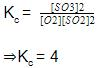Courses

# Test: Homogeneous Equilibrium(Chemical Equilibrium)

## 21 Questions MCQ Test Chemistry Class 11 | Test: Homogeneous Equilibrium(Chemical Equilibrium)

Description
This mock test of Test: Homogeneous Equilibrium(Chemical Equilibrium) for JEE helps you for every JEE entrance exam. This contains 21 Multiple Choice Questions for JEE Test: Homogeneous Equilibrium(Chemical Equilibrium) (mcq) to study with solutions a complete question bank. The solved questions answers in this Test: Homogeneous Equilibrium(Chemical Equilibrium) quiz give you a good mix of easy questions and tough questions. JEE students definitely take this Test: Homogeneous Equilibrium(Chemical Equilibrium) exercise for a better result in the exam. You can find other Test: Homogeneous Equilibrium(Chemical Equilibrium) extra questions, long questions & short questions for JEE on EduRev as well by searching above.
QUESTION: 1

Solution:
QUESTION: 2

### On taking 60.0 g CH3COOH and 46.0 g CH3CH2OH in a 5 L flask in the presence of H30+ (catalyst), at 298 K 44.0 g of CH3COOC2H5 is formed at equilibrium. If amount of CH3COOH is doubled without affecting amount of CH3CH2OH, then CH3COOC2H5 formed is

Solution:

Molar mass of CH3COOH=60gmol-1
Molar mass of C2H5OH=46gmol-1
Molar mass of CH3COOC2H5=88gmol-1
∴[CH3COOH)Initial=6060×5=0.2molL-1
[C2H5OH]Initial=4646×5=0.2molL-1
[CH3COOC2H5]eqm=4488×15=0.1molL-1
CH3COOH+C2H5OH⇔CH3COOC2H5+H2O
Initial
0.2M          0.2M
0.2M           0.2M
At eqm.
(0.2−0.1)M (0.2−0.1)M 0.1M 0.1M
(0.2-0.1)M (0.2-0.1)M 0.1M 0.1M
∴K=[CH3COOC2H5][H2O]/[CH3COOH][C2H5OH]
=0.1×0.1/0.1×0.1=1
In second case,
[CH3COOH]Initial=0.4M
[C2H5OH]Initial=0.2M
If x is the amount of acid and alcohol reacted
[CH3COOH]eqm.=(0.40-x)M
[C2H5OH]eqm.=[0.2-x]M
[CH3COOC2H5]eqm.=[H2O]eqm.=xM
∴K=x2/(0.4-x)(0.2-x)=1
x=860M
∴Moles of ethyl acetate produced =860×5=23
Mass of ethyl acetate produced =23×88=58=58.66g.

QUESTION: 3

### For the reversible reaction,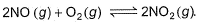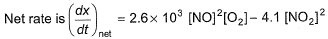In a reaction vessel, [NO]= [O2]= 0.01 mol L-1 and [NO2]= 0.1 mol L-1 then above reaction is

Solution:

On substituting the values of conc. of NO, O2 and NO2 in given rate equation, we get a +ve (positive) value indicating that the reaction takes place in forward direction.

QUESTION: 4

The equilibrium constant for the following reaction, is 1.6 x 105 at 1024 K.

H2(g) + Br2(g) 2HBr(g)
HBr (g)at 10.0 bar is introduced into a sealed container at 1024 K. Thus, partial pressure of H2(g)and Br2(g), together is

Solution: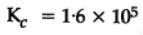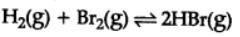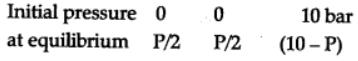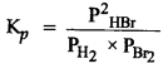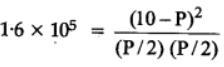Squaring on both sides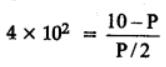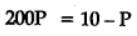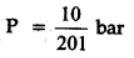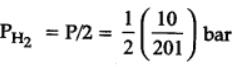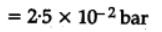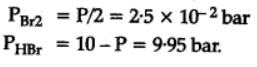=> 10 bar approximately

QUESTION: 5

At 273 K and 1 atm, 1 L of N2O4 (g) decomposes to NO2(g)a s given,

N2O4(g) ⇌2NO2(g)

At equilibrium, original volume is 25% less than the existing volume. Percentage decomposition of N2O4 (g) is thus,

Solution:

Let the initial volume of N2O4 be x and initial volume of NO2 is 0
If the degree of dissociation is a, then the final volume of N2O4 is x(1−a) and NO2 is 2ax.
Initial
It equilibrium
N2O4            ⟶              2NO2
x                                       0
x(1−a)                               2ax
Total initial volume =x+0=x
Final volume =x(1−a)+2ax=x+ax=x(1+a)
It is given that the initial volume is 25% less than the final volume
x=0.75×(1+a)
1+a=1.33
a=0.33
So %age dissociation = 33.33%

QUESTION: 6

H2S (g) initially at a pressure of 10.0 atm and a temperature of 800 K, dissociates as

2H2S(g) ⇌ 2H2(g) + S2(g)

At equilibrium, the partial pressure of S2 vapour is 0.020 atm . Thus, Kp is

Solution:

The correct answer is Option A.

2H2S(g) ⇌ 2H2(g) + S2(g)

Pressure
at t=0           Pi                −           −
at eqm       Pi−P            2P          P

as P=0.02    thus Pi−P=10−0.02
Pi=10                     2P=0.04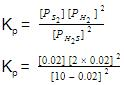Kp = 3.23×10−7 atm.

QUESTION: 7

A sample of N2O4(g)with a pressure of 1.00 atm is placed in a flask. When equilibrium is reached, 20% of N2O4(g)has been converted to NO2(g)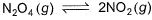If the original pressure is made 10% of the earlier pressure, then per cent dissociation will be

Solution:

Correct answer is A.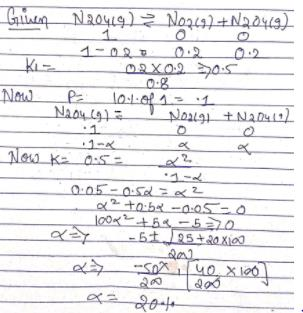QUESTION: 8

Following equilibrium is set up at 1000 K and 1 bar in a 5 L flask,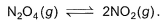At equilibrium, NO2 is 50% o f the total volume. Thus, equilibrium constant Kc is

Solution:

The correct answer is Option A.
N2O4  ⇌  2NO2
Initial            1                 0
Equilibrium  1−x             2x

Total moles = 1 - x + 2x

NO2 is 50% of the total volume when equilibrium is set up.
Thus, the volume fraction (at equilibrium) of NO2 = 50/100 = 0.5 = ½
So,    2x / (1+x) = ½
=> x = ⅓

For 1 litre;
Kc = [NO2] / [N2O4]
= [4*(1/9)] / [⅔]
= 0.66;

For 5 litres;
Kc = 0.66 / 5
= 0.133
Thus, option A is correct.

QUESTION: 9

Following equilibrium is set up at 298 K in a 1 L flask.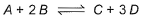If one starts with 2 moles of A and 1 mole of B, it is found that moles of B and D are equal.Thus Kc is

Solution:

For the equilibrium reaction:
A+2B ⇌ 2C+D
volume of flask = 1L
Initial moles of A = 2 mol
initial concentration of A=[A]i = 2 M
initial mole of B = 1 mol
[B]i = 1 M
[A]eq = 2-x, [B]eq = 1-2x, [C]eq = x, [D]eq = 3x
Given [D]eq = 1 * 1L
= 1 M
Thus x = 1M
[A]eq = 1, [B]eq = -1, [C]eq = 1, [D] = 3
Kc = {([D]eq)3 * ([C]eq)}/{[A]eq * ([B]eq)2
= Kc = {(3)3*1}/{1*(-1)2}
= 27/1
= 27

QUESTION: 10

At 700 K and 350 bar, a 1 : 3 mixture of N2(g) and H2(g) reacts to form an equilibrium mixture containing X (NH3)= 0.50. Assuming ideal behaviour Kp for the equilibrium reaction,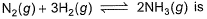Solution:

The correct answer is option A
2.03x 10-4
The given equation is :-
N2​(g)+3H2​(g) ⇌ 2NH3​(g)
Initial moles : 1             3         0
At eqm ;       (1−x)    (3−3x)   (2x)
(let)
Total moles of equation
=1 − x + 3 − 3x + 2x = (4−2x)
Now, X(NH3​) =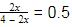⇒ 2x = 2 − x
⇒ 3x = 2 ⇒ x = 0.66 =
32​
Now, at equation, moles of N2​= 1/3, moles of NH3​ = 4/3
moles of H2 ​ =3 − 2 = 1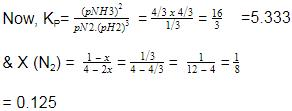QUESTION: 11

Equilibrium constant for the reaction,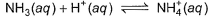is 1.8 x 109. Hence, equilibrium constant for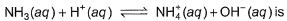Solution:

The correct answer is Option A.

NH4OH + H+ ⇌ NH4+ + H2​O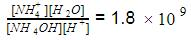Again,
NH3 + H2O → NH4OH ⇌ NH
4++ OH-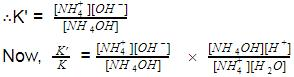= [OH
-] [H+] (∵H2​O is in excess)
=Kw
=1×10-14
∴K = K×1×10-14
=1.8×109×10-14
=1.8×10-5

QUESTION: 12

A gaseous phase reaction taking place in 1L flask at 400 K is given,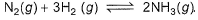Starting with 1 mole N2 and 3 moles H2, equilibrium mixture required 250 mL of 1M H2SO4 . Thus, Kc is

Solution:
QUESTION: 13

For the following equilibrium starting with 2 moles SO2 and 1 mole O2 in 1 L flask,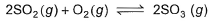Equilibrium mixture required 0.4 mole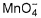in acidic medium. Hence, Kc is

Solution:

0.4m of KMnO4 = 1 mole of SO2
​= 1 mole of SO
2SO2 + O2 ⇌ 2SO3
2      1         0
1      0.5       1
K = [SO3]2/[SO2]2 [O2]
= 12/(12*0.5)
= 2

QUESTION: 14

Direction (Q. Nos. 14 and 15) This sectionis based on statement I and Statement II. Select the correct answer from the code given below.

Q. Statement I

If Kc = 4 for the following equilibrium,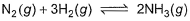Then mixture of 1 mole N2, 3 moles H2 and 2 moles NH3 in 1L flask is in equilibrium.

Statement II

Reaction quotient of the given quantities is less than the equilibrium constant.

Solution:
QUESTION: 15

Statement I

1 mole A(g) and 1 mole B(g)give 0.5 mole of C(g)and 0.5 mole D(g) at equilibrium.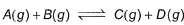On taking 2 moles each of A(g)and B(g), percentage dissociation A(g)and B(g) is also doubled.

Statement II

Equilibrium constant, Kc = 1

Solution:
QUESTION: 16

Direction (Q. Nos. 16-19) This section contains  a paragraph, each describing theory, experiments, data etc. three Questions related to paragraph have been given.Each question have only one correct answer among the four given options (a),(b),(c),(d)

Passage I
The equilibrium reaction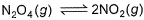has been thoroughly studied Kp = 0.148 at 298 K

If the total pressure in a flask containing NO2 and N2O4 gas at 25°C is 1.50 atm, what fraction of the N2O4 has dissociated to NO2

Solution:

The correct answer is Option A.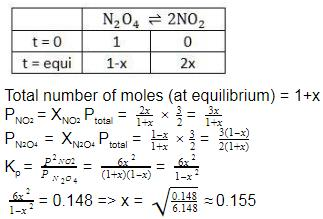Fraction of N2O4 dissociated = x = 0.155 (x = mole fraction)

QUESTION: 17

Passage I
The equilibrium reactionhas been thoroughly studied Kp = 0.148 at 298 K

Q. If the volume of the container is increased so that the total equilibrium pressure falls to 1.00 atm, then fraction of N2O4 dissociated is

Solution:
QUESTION: 18

Passage II

A 15 L flask at 300 K contains 64.4 g of a mixture of NO2 and N2O4 in equilibrium. Given,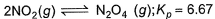Q. Kc for the above equilibrium is

Solution:

Kp = Kc(RT)n

Kp = 6.67 ,
∆n = moles of products - moles of reactants = 1-2 = -1
R = 0.0821 L atm mol-¹K-¹
T = 300K

Substitute these values in the formula,
=> Kc = 6.67×0.0821×300
Kc = 164.28.

QUESTION: 19

Passage II

A 15 L flask at 300 K contains 64.4 g of a mixture of NO2 and N2O4 in equilibrium. Given,Q. Total pressure in the flask is

Solution:
QUESTION: 20

Direction (Q. Nos. 20) Choice the correct combination of elements and column I and coloumn II  are given as option (a), (b), (c) and (d), out of which ONE option is correct.

Q. In the following equilibrium,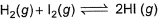is set up at 298 K in a 5 L flask. Experiment is carried by taking 0.1 mole each of H2(g)and l2(g). After equilibrium is attained, l2(g)was dissolved in Kl and required 100 mL of 0.1 M Na2S2O3 solution.

Match the values given in Column II with the respective terms in Column I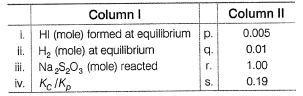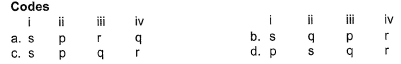Solution:
*Answer can only contain numeric values
QUESTION: 21

Direction (Q. Nos. 21) This section contains 2 questions. when worked out will result in an integer from 0 to 9 (both inclusive)

Q. For the equilibrium in gaseous phase in 2 L flask we start with 2 moles of SO2 and 1 mole of O2 at 3 atm,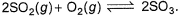When equilibrium is attained, pressure changes to 2.5 atm. Hence, equilibrium constant Kc is

Solution:

The correct answer is 4
2SO2(g) + O2(g) ⇋ 2SO3
Initial moles      2            1
At equilibrium 2 - 2x     1 - x    2x
Net moles at equilibrium  =  2 - 2x + 1 - x + 2x
=(3 - x)moles
Initial:
moles = 3,
Pressure = 3 atm,
Volume = 2L,
PV = nRT
3 x 2 = 3RT  -------- 1
At equilibrium
Moles = 3 - x,
Pressure = 2.5 atm
Volume = 2L
P‘V = n’RT ---------- 2
Divide eqn  2 by 1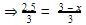⇒2.5 = 3 - x
⇒x = 0.5